## 中间层厚度对三明治结构中 I 型断裂能量释放率的影响研究$^{\bf 1)}$

*中国科学院力学研究所非线性力学国家重点实验室,北京 100190

**北京大学工学院,北京 100871

## EFFECT OF INTERLAYER THICKNESS ON THE MODE I FRACTURE ENERGY RELEASE RATE OF SANDWICH STRUCTURE$^{\bf 1)}$

Zhang Fanfan*,, Song Jingru*,, Ma Hansong*,, Liu Xiaoming,*,,2), Wei Yueguang**

*LNM,Institute of Mechanics,Chinese Academy of Sciences, Beijing 100190,China

School of Engineering Science,University of Chinese Academy of Sciences,Beijing 100049,China

**College of Engineering,Peking University,Beijing 100871, China

 基金资助: 1)国家基础科学中心.  11988102国家自然科学基金.  11772334国家自然科学基金.  11972347国家自然科学基金.  11432014中国科学院先导B基金.  XDB22040501

Received: 2020-03-25   Accepted: 2020-06-4   Online: 2020-07-18Abstract

As a load transfer and connection element, the sandwich structure is widely used in aerospace, material characterization, flexible electronics and other fields. Understanding its fracture behavior and characteristics can provide theoretical guidance for designing the load capacity of the sandwich structure connector. In this paper, based on the improved elastic foundation theoretical model, we proposed a new theoretical model to calculate the energy release rate of the sandwich structure. The theoretical model considered the effect of the interlayer thickness on the energy release rate of the mode I fracture energy of the sandwich structure. Results showed that the influence of the middle layer on the energy release rate of mode I fracture has two parts: the influence of the shear force of the middle layer and the effect of the middle layer on the increase of structural stiffness. When the dimensionless interlayer thickness takes the maximum value of 2, the energy release rate from the traditional model may have a deviation greater than 70%, compared with the finite element calculation; our model can greatly improve the accuracy, and the error can be reduced to 5%. Compared with the improved elastic foundation theory, which is only applicable to the case where the thickness of the middle layer is small, the theoretical model has a wider range of applications. In addition, by using the present model, two geometric parameters (intermediate layer thickness and initial crack length) and one material parameter (modulus ratio) were selected for the study. The sensitivity of shear effect to structural geometry and material parameters was discussed. Based on the constant load, the influence of geometric and material parameters on the energy release rate was discussed; and on the assumption that the fracture toughness of the structure remains unchanged, the influence law of geometric and material parameters on the critical load of the sandwich structure was obtained.

Keywords： sandwich structure ; interlayer influence ; interface shear ; energy release rate ; critical load

Zhang Fanfan, Song Jingru, Ma Hansong, Liu Xiaoming, Wei Yueguang. EFFECT OF INTERLAYER THICKNESS ON THE MODE I FRACTURE ENERGY RELEASE RATE OF SANDWICH STRUCTURE$^{\bf 1)}$. Chinese Journal of Theoretical and Applied Mechanics[J], 2020, 52(4): 1080-1094 DOI:10.6052/0459-1879-20-092

## 引言

Ouezdou 等提出的理论模型是研究三明治结构断裂韧性的一个经典模型,很多文献将其作为参考来研究纯 I 型模式下三明治结构的断裂韧性[21-28],本文将其称为改良弹性地基理论模型. 改良弹性地基理论模型是通过考虑有限长度的梁而获得的,如图 1 所示. 三明治结构关于中间层的中心线轴对称,$x <0$ 的区域用欧拉-伯努利梁理论进行求解,$x >0$ 的区域用 Winkler 地基模型进行求解,最后通过两个区域的连续性等条件求得结构的能量释放率. 已有发现,中间层厚度较小的时候,该模型可以较好地预测三明治结构的断裂韧性[21-28]. 但是,当中间层厚度较大的时候,该模型与实验结果有一定的偏差. 通常情况下,三明治结构的中间层厚度较小,在0.1$\sim$2.0mm 之间[29-30]. 近期,随着大型造船结构等的兴起,中间层厚度较大 (10mm 左右) 的三明治结构相继出现,然而目前有关中间层较厚的三明治结构的相关研究还很少. 所以,研究不同中间层厚度对三明治结构断裂行为的影响具有重要的科学和工程意义.

## 1 模型描述

### 图1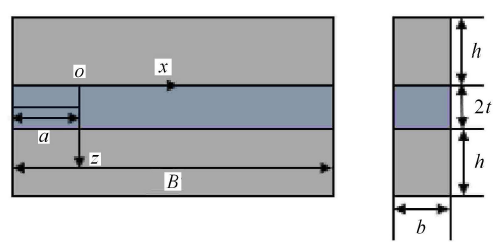Fig.1   Sandwich structure diagram

### 图2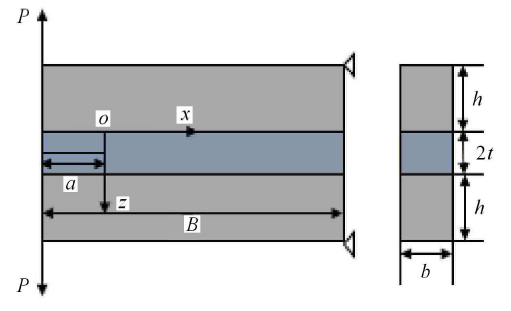Fig.2   Load diagram of sandwich structure

### 1.2 改良弹性地基理论模型及其局限性

Table 1  Material and geometric parameters of finite element model 1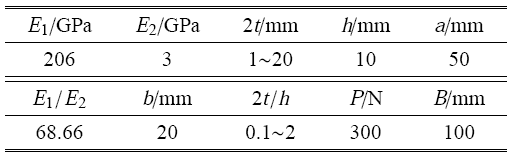### 图3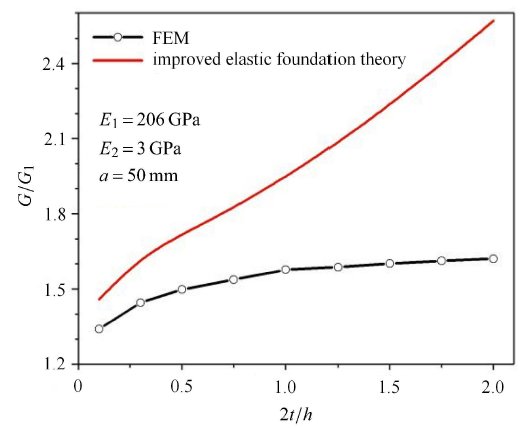Fig.3   Variation of dimensionless energy release rate with dimensionless interlayer thickness—Comparison of improved elastic foundation theoretical model and finite element simulation

1.3.1 新模型建立的依据

### 图4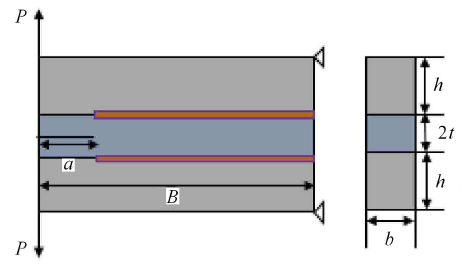Fig.4   Schematic diagram of finite element model 2

Table 2  Material and geometric parameters of finite element model 2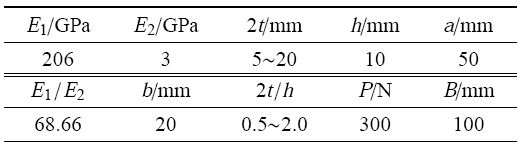### 图5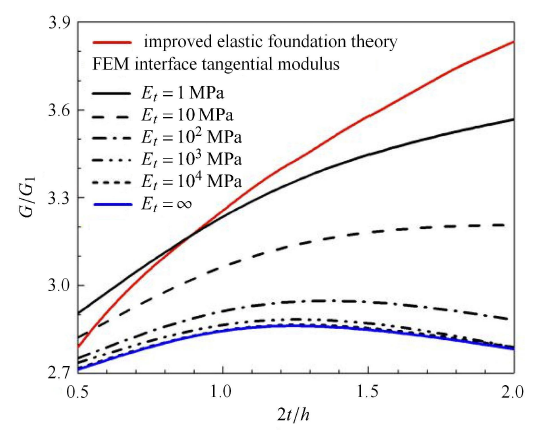Fig.5   Variation of dimensionless energy release rate with dimensionless interlayer thickness at different interface tangential modulus

1.3.2 引入中间层剪切力的影响

$u_{(1x^ - )} = u_{(1x^ - 0)} - \left(z + \dfrac{h}{2}\right)\dfrac{\text{d}w_{(1x^ - )} }{\text{d}x}$
$w_{(1x^ - )} = w_{(1x^ - )} \left( x \right)$

$x<0$ 区域长度为 $\text{d}x$ 的非中间层微元体受力图如图 6 所示.

### 图6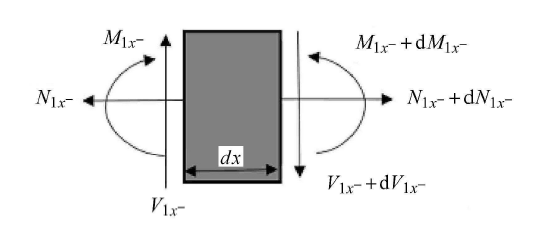Fig.6   Non-interlayer force balance diagram of microelements with length $\text{d}x$

$\left.\begin{array}{c} \dfrac{\text{d}N_{(1x^ - )} }{\text{d}x} = 0 \\ \dfrac{\text{d}V_{(1x^ - )} }{\text{d}x} = 0 \\ \dfrac{\text{d}M_{(1x^ - )} }{\text{d}x} = V_{(1x^ - )} \end{array} \right \}$

$N_{(1x^ - )} = \int_{(A_{(1x^ - )} )} {(\sigma _{xx} )_{(1x^ - )} } \text{d}A = E_1 A_{(1x^ - )} \dfrac{\text{d}u_{(1x^ - 0)} }{\text{d}x}$
$M_{(1x^ - )} = \int_{(A_{(1x^ - )} )} {(\sigma _{xx} )_{(1x^ - )} } \left(z + \dfrac{h}{2} \right ) \text{d}A = - E_1 I_{(1x^ - )} \dfrac{\text{d}^2w_{(1x^ - )} }{\text{d}x^2}$

$\left.\begin{array}{c} E_1 A_{(1x^ - )} \dfrac{\text{d}^2u_{(1x^ - 0)} }{\text{d}x^2} = 0 \\ E_1 I_{(1x^ - )} \dfrac{\text{d}^4w_{(1x^ - )} }{\text{d}x^4} = 0 \end{array} \right \}$

$u_{(1x^ + )} = u_{(1x^ + 0)} - (z + \dfrac{h}{2})\dfrac{\text{d}w_{(1x^ + )} }{\text{d}x}$
$w_{(1x^ + )} = w_{(1x^ + )} \left( x \right)$

$u_2 = a_1 z^2 + b_1 z + c_1$

### 图7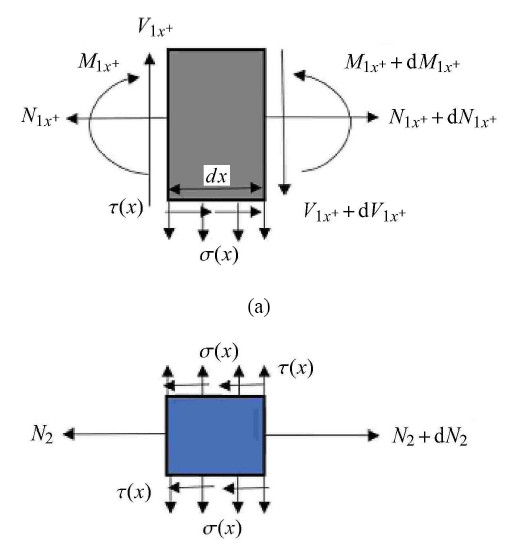Fig.7   Non- interlayer (a) and interlayer (b), the force balance diagram of microelements with length $\text{d}x$

$x >0$ 区域长度为 $\text{d}x$ 的中间层和非中间层的微元体受力图如图所示.

$\left.\begin{array}{c} \dfrac{\text{d}N_{(1x^ + )} }{\text{d}x} = - \tau (x)b \\ \dfrac{\text{d}V_{(1x^ + )} }{\text{d}x} = - \sigma (x)b \\ \dfrac{\text{d}M_{(1x^ + )} }{\text{d}x} = V_{(1x^ + )} - \tau (x)\dfrac{bh}{2} \end{array} \!\! \right \}$

$\dfrac{\text{d}N_2 }{\text{d}x} = 2\tau (x)b$

$N_{(1x^ + )} = \int_{(A_{(1x^ + )} )} {(\sigma _{xx} )_{(1x^ + )} } \text{d}A = E_1 A_{(1x^ + )} \dfrac{ \text{d}u_{(1x^ + 0)} }{\text{d}x}$
$N_2 = \int_{(A_2 )} (\sigma _{xx} )_2 \text{d}A = bE_2 \Bigg (\dfrac{\text{d}a_1 }{\text{d}x}\dfrac{8t^3}{3} + \dfrac{\text{d}b_1 }{\text{d}x}2t^2 + \dfrac{\text{d}c_1 }{\text{d}x}2t \Bigg )$
$M_{(1x^ + )} = \int_{(A_{(1x^ + )} )} (\sigma _{xx} )_{(1x^ + )} \Bigg(z + \dfrac{h}{2} \Bigg) \text{d}A = - E_1 I_{(1x^ + )} \dfrac{\text{d}^2w_{(1x^ + )} }{\text{d}x^2}$

$E_1 A_2 \dfrac{ \text{d}u_{(1x^ + 0)} }{ \text{d}x} = - \tau(x)b$
$- E_1 I_{(1x^ + )} \dfrac{ \text{d}^4w_{(1x^ + )} }{\text{d}x^4} = - \sigma(x)b - \dfrac{\text{d}\tau (x)}{ \text{d}x}\dfrac{bh}{2}$
$bE_2 \Bigg (\dfrac{\text{d}^2a_1 }{\text{d}x^2}\dfrac{8t^3}{3} + \dfrac{\text{d}^2b_1 }{\text{d}x^2}2t^2 + \dfrac{\text{d}^2c_1 }{\text{d}x^2}2t \Bigg ) = 2\tau (x)b$

$\sigma (x) = - kw_{(1x^ + )} = - \dfrac{E_2 }{t}w_{(1x^ + )}$

$z = 0, \ \ u_2 = u_{(1x^ + )}$

$z = 0, \ \ \tau (x) = G\dfrac{\partial u_2 }{\partial z}$

$z = t, \ \ \tau (x) = G\dfrac{\partial u_2 }{\partial z} = 0$

$\dfrac{2(1 + v_2 )}{9}\dfrac{E_1 }{E_2 }t^2h^2\dfrac{\text{d}^6w_{(1x^ + )} }{\text{d}x^6} - \Bigg(\dfrac{4}{3}th + \dfrac{h^2}{3}\dfrac{E_1 }{E_2 }\Bigg )\dfrac{\text{d}^4w_{(1x^ + )} }{\text{d}x^4} + \\ \qquad \dfrac{8t(1 + v_2 )}{3h}\dfrac{\text{d}^2w_{(1x^ + )} }{\text{d}x^2} - \Bigg(\dfrac{4}{h^2}\dfrac{E_2 }{E_1 } + \dfrac{4}{ht}\Bigg ) w_{(1x^ + )} = 0$
$b_1 = \dfrac{\dfrac{E_1 }{9G}t^2h^2\dfrac{\text{d}^5w_{(1x^ + )} }{\text{d}x^5} - th\dfrac{\text{d}^3w_{(1x^ + )} }{\text{d}x^3} + \dfrac{4tE_2 }{3Gh}\dfrac{\text{d}w_{(1x^ + )} }{\text{d}x}}{2G\Bigg(\dfrac{1}{E_2 } + \dfrac{t}{hE_1 }\Bigg )}$
$a_1 = \dfrac{ - b_1 }{2t}$
$u_{(1x^ + 0)} - \dfrac{h}{2}\dfrac{\text{d}w_{(1x^ + )} }{\text{d}x} = c_{1 }$
$E_1 A_{(1x^ + )} \dfrac{\text{d}u_{(1x^ + 0)} }{\text{d}x} = - \tau(x)b$
$hE_1 \dfrac{\text{d}^2u_{(1x^ + 0)} }{\text{d}x^2} = - Gb_1$

$hE_1 \dfrac{\text{d}^2u_{(1x^ + 0)} }{\text{d}x^2} = - Gb_1$

$hE_1 \dfrac{\text{d}^2u_{(1x^ + 0)} }{\text{d}x^2} = - Gb_1$
$\dfrac{2(1 + v_2 )}{9}\dfrac{E_1 }{E_2 }t^2h^2\dfrac{\text{d}^6w_{(1x^ + )} }{\text{d}x^6} - \Bigg(\dfrac{4}{3}th + \dfrac{h^2}{3}\dfrac{E_1 }{E_2 }\Bigg )\dfrac{\text{d}^4w_{(1x^ + )} }{\text{d}x^4} +\\ \qquad \dfrac{8t(1 + v_2 )}{3h}\dfrac{\text{d}^2w_{(1x^ + )} }{\text{d}x^2} - \Bigg (\dfrac{4}{h^2}\dfrac{E_2 }{E_1 } + \dfrac{4}{ht}\Bigg )w_{(1x^ + )} = 0$

$\left.\begin{array}{c} E_1 A_{(1x^ - )} \dfrac{\text{d}^2u_{(1x^ - 0)} }{\text{d}x^2} = 0 \\ E_1 I_{(1x^ - )} \dfrac{\text{d}^4w_{(1x^ - )} }{\text{d}x^4} = 0 \end{array} \!\! \right \}$

$\left. \begin{array}{c} u_{(1x^ - 0)} = m_1 x + m_2 \\ w_{(1x^ - )} = Ax^3 + Bx^2 + Cx + D \end{array} \right \}$

$hE_1 \dfrac{\text{d}^2u_{(1x^ + 0)} }{\text{d}x^2} = - Gb_1$
$\dfrac{2(1 + v_2 )}{9}\dfrac{E_1 }{E_2 }t^2h^2\dfrac{\text{d}^6w_{(1x^ + )} }{\text{d}x^6} - \Bigg(\dfrac{4}{3}th + \dfrac{h^2}{3}\dfrac{E_1 }{E_2 } \Bigg )\dfrac{\text{d}^4w_{(1x^ + )} }{\text{d}x^4} +\\ \qquad \dfrac{8t(1 + v_2 )}{3h}\dfrac{\text{d}^2w_{(1x^ + )} }{\text{d}x^2} - \Bigg(\dfrac{4}{h^2}\dfrac{E_2 }{E_1 } + \dfrac{4}{ht}\Bigg)w_{(1x^ + )} = 0$

$u_{(1x^ + 0)} = \int \Bigg \{ \int \Big [ - (Gb_1 ) / (hE_1 )\Big ] \text{d}x + n_1 \Bigg \} \text{d}x + n_2$

$\dfrac{2(1 + v_2 )E_1 t^2h^2}{9E_2 }r^6 - \Bigg (\dfrac{4}{3}th + \dfrac{h^2}{3}\dfrac{E_1 }{E_2 }\Bigg)r^4 +\\ \qquad \dfrac{8t(1 + v_2 )}{3h}r^2 - \Bigg (\dfrac{4E_2 }{h^2E_1 } + \dfrac{4}{ht}\Bigg) = 0$

(1) 当其特征根全为实根时,解为

$w_{(1x^ + )} = C_1 {\rm e}^{( - r_1 x)} + C_2 {\rm e}^{( - r_2 x)} + C_3 {\rm e}^{( - r_3 x)} +\\ \qquad C_4 {\rm e}^{r_1 x} + C_5 {\rm e}^{r_2 x} + C_6 {\rm e}^{r_3 x}$

(2) 当其特征根为一实根两虚根时,解为

$w_{(1x^ + )} = C_1 {\rm e}^{( - r_1 x)} + {\rm e}^{( - r_2 x)}(C_2 \cos(r_3 x) + C_3 \sin(r_3 x)) + \\ \qquad C_4 {\rm e}^{r_1 x} + {\rm e}^{r_2 x}(C_5 \cos(r_3 x) + C_6 \sin(r_3 x)$

$V_{1x^ - }(x = - a,z = - h ) = P$
$M_{1x^ - } \Bigg(x = - a,z = - \dfrac{h}{2} \Bigg) = 0$
$N_{1x^ - } \Bigg(x = - a,z = - \dfrac{h}{2} \Bigg) = 0$
$N_{1x^ + } \Bigg(x = B - a,z = - \dfrac{h}{2} \Bigg) = 0$
$u_{1x^ + }(x = B - a,z = - h) = 0$
$w_{1x^ - }(x = 0) = w_{1x^ + } (x = 0)$
$\left. {\dfrac{\text{d}w_{1x^ - } }{\text{d}x}} \right|_{(x = 0)} = \left. {\dfrac{\text{d}w_{1x^ + } }{\text{d}x}} \right|_{(x = 0)}$
$V_{1x^ - }(x = 0) = V_{1x^ + } (x = 0)$
$M_{1x^ - }(x = 0) = M_{1x^ + } (x = 0)$
$u_{1x^ - }(x = 0) = u_{1x^ + } (x = 0)$
$N_{1x^ - }(x = 0) = N_{1x^ + } (x = 0)$

(a) 左端 ($x=-a$) 处剪力为 $P$;

(b) 左端 ($x=-a$) 弯矩为 0;

(c) 左端 ($x=-a$) 轴力为 0;

(d) 右端 ($x=B-a$) 轴力为 0;

(e) 右端最上端 ($x=B-a)u=0$;

(f) 中间 $(x=0)$ 挠度连续;

(g) 中间 $(x=0)$ 转角连续;

(h) 中间 $(x=0)$ 剪力连续;

(i) 中间 $(x=0)$ 弯矩连续;

(j) 中间 $(x=0)$ 位移 $u$ 连续;

(k) 中间 $(x=0)$ 轴力连续.

$C = \left. {\dfrac{2w_{(1x^ - )} (x)}{P}} \right|_{x = - a}$

$G = 2\dfrac{P^2}{2b}\dfrac{\text{d}C}{\text{d}a}$

1.3.3 引入中间层厚度增大带来的结构刚度增大的影响

### 图8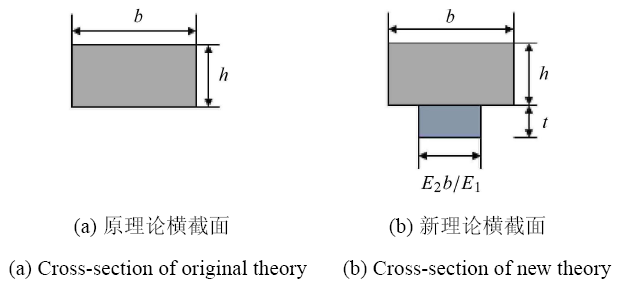Fig.8   Schematic cross-section of the original theory and the theory considering the effect of increased stiffness

$h_{\rm eff} = \sqrt{\dfrac{12I_1 }{b}}$

$I_1$ 表示新截面即新理论模型横截面中性轴的惯性矩. 然后,通过减去 $x <0$ 区域只考虑非中间层的悬臂梁贡献的能量释放率 (即 1.2 中提到的 $G_{1}$),加上将非中间层和中间层都考虑的等效复合悬臂梁贡献的能量释放率,得到同时引入中间层剪切力的影响和中间层厚度增大带来的结构刚度增大的影响的三明治结构能量释放率表达式

$G = 2\dfrac{P^2}{2b}\dfrac{\text{d}C}{\text{d}a} - \dfrac{12P^2a^2}{E_1 h^3b^2} + \dfrac{12P^2a^2}{E_1 h_{\rm eff}^3 b^2}$

### 1.4 改良弹性地基理论模型与新模型的对比

(1) 不同中间层厚度下,改良弹性地基模型与新模型的对比.

### 图9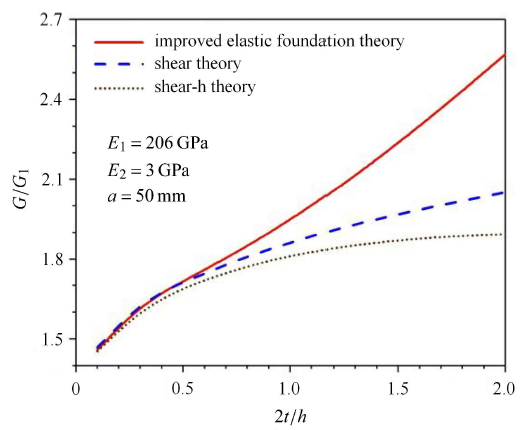Fig.9   Variation of dimensionless energy release rate with dimensionless interlayer thickness—Comparison of theoretical models

(2) 不同模量比下,改良弹性地基模型与新模型的对比. 本节研究不同模量比下,改良弹性地基模型与新模型的对比.由上节可知,中间层厚度的范围也决定了新引入量能否对能量释放率产生影响. 所以,本节分别研究无量纲中间层厚度取最小值 $(2t/h=0.1)$ 和无量纲中间层厚度取最大值 $(2t/h=2)$ 时,新引入量对能量释放率产生影响的模量比范围. 其他参数取值为：取总长 $B$ 为 100mm,取 $y$ 方向上的宽度 $b$ (垂直于图平面) 为 20mm,裂纹初始长度 $a$ 为 50mm,非中间层厚度 $h$ 为 10mm. 假设中间层为环氧胶,取其材料参数为：弹性模量 $E_{2}=3$GPa,泊松比 $v_{2} =0.3$;假设非中间层的弹性模量 $E_{1}$ 取 60$\sim$300GPa,泊松比 $v_{1} =0.3$. 本文定义非中间层模量 $E_{1}$ 与中间层模量 $E_{2}$ 的比值为模量比,记作 $E_{1}/E_{2 }$,取 20$\sim$100. 取载荷 $P$ 为 300N. 将模量比 $E_{1}/E_{2}$ 作为横坐标;将无量纲能量释放率 $G/G_{1}$ 作为纵坐标.

### 图10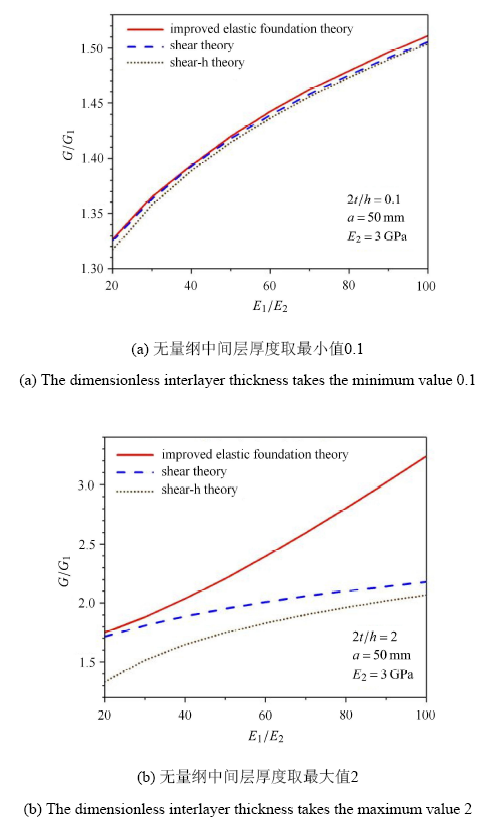Fig.10   Variation of dimensionless energy release rate with modulus ratio—Comparison of theoretical models

(3) 不同裂纹初始长度下,改良弹性地基模型与新模型的对比. 本节研究不同裂纹初始长度下,改良弹性地基模型与新模型的对比. 和上节一样,本节分别研究无量纲中间层厚度取最小值 $(2 t/h =0.1)$ 和无量纲中间层厚度取最大值 $(2 t/h =2)$ 时,新引入量产生影响的裂纹初始长度范围. 其他参数取值为：取总长 $B$ 为 100mm,取 $y$ 方向上的宽度 $b$ (垂直于图平面)为 20mm,取裂纹初始长度 $a$ 为 20$\sim$50mm,取非中间层厚度 $h$ 为 10mm. 假设非中间层为钢材,取其材料参数为：弹性模量 $E_{1} =206$GPa,泊松比 $v_{1} =0.3$;假设中间层为环氧胶,取其材料参数为：弹性模量 $E_{2}=3$GPa,泊松比 $v_{2} =0.3$. 取载荷 $P$ 为 300N. 本文定义裂纹初始长度 $a$ 与非中间层厚度 $h$ 的比值为无量纲裂纹初始长度,记作 $a/h$,将其作为横坐标;将无量纲能量释放率 $G/G_{1}$ 作为纵坐标.

### 图11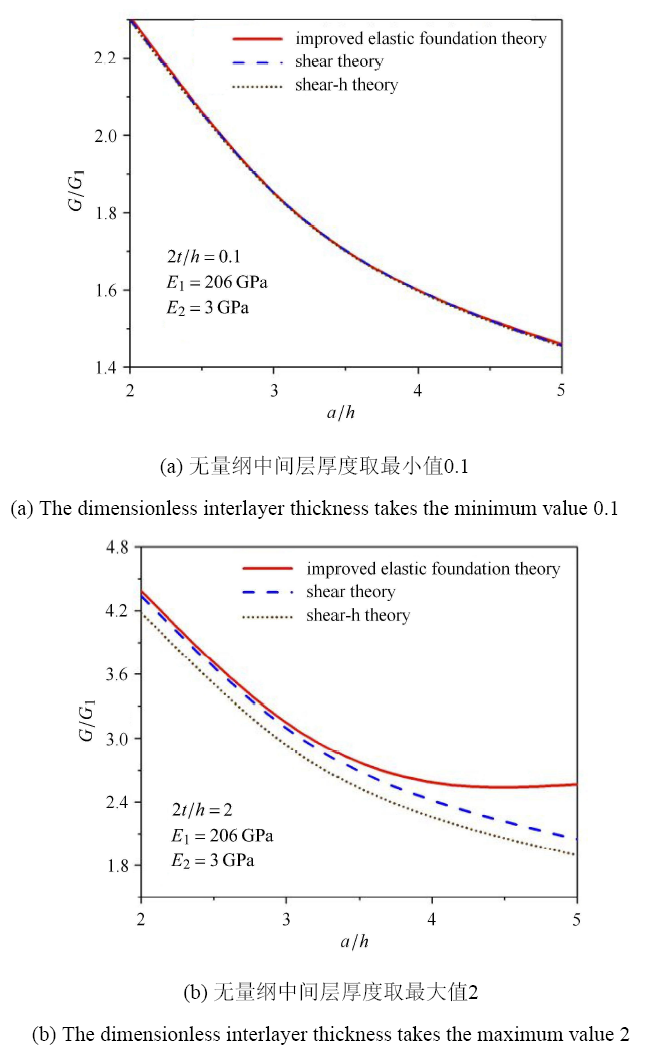Fig.11   Variation of dimensionless energy release rate with initial length of dimensionless crack—Comparison of theoretical models

## 2 几何参数和材料参数对能量释放率和临界载荷的影响

### 图12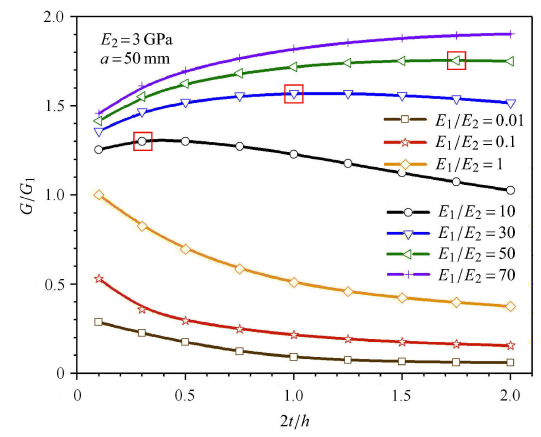Fig.12   Variation of dimensional energy release rate with dimensionless interlayer thickness and modulus ratio

### 图13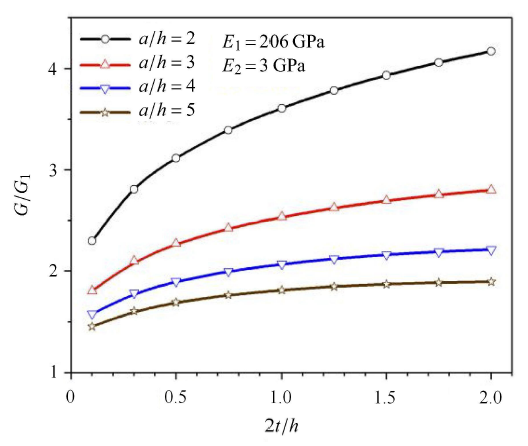Fig.13   Variation of dimensional energy release rate with dimensionless interlayer thickness and dimensionless crack initial length

### 图14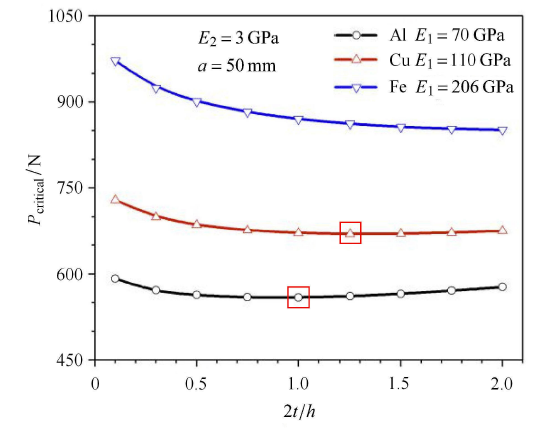Fig.14   Variation of critical load with dimensionless interlayer thickness and modulus ratio

### 图15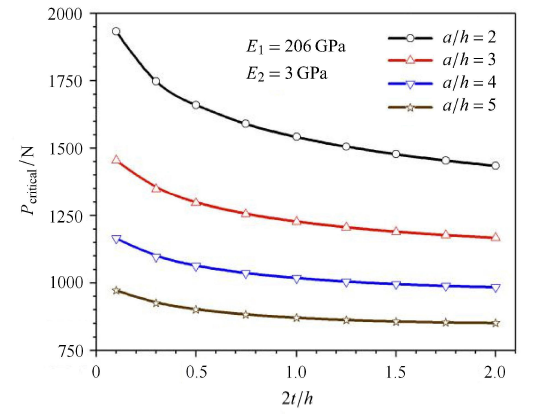Fig.15   Variation of critical load with dimensionless interlayer thickness and dimensionless crack initial length

## 3 讨论

### 图16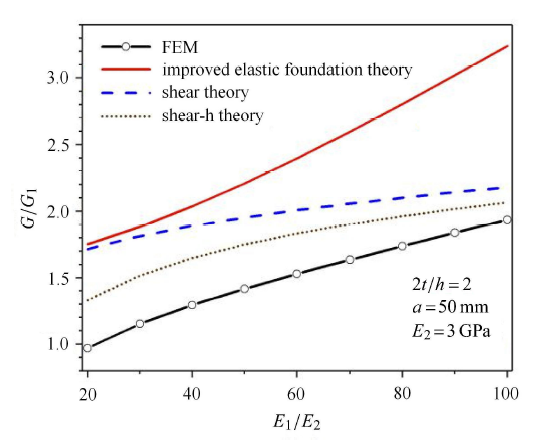Fig.16   Variation of dimensionless energy release rate with modulus ratio—Comparison of theoretical models and finite element simulation

## 4 结论

(1) 建立了同时考虑中间层剪切影响和刚度影响的三明治结构断裂模型,通过和有限元计算结果比较,预测的 I 型裂纹能量释放率比已有理论模型更精确.

(2) 相同的载荷下计算获得的能量释放率与模量比有关：当三明治结构中间层的模量小于边上两层模量时,能量释放率随着中间层厚度增大先增大后减小;当三明治结构中间层的模量大于边上两层模量时,能量释放率仅随着中间层厚度增大而减小.

(3) 对于常见的金属 (铁/铜/铝) 和环氧胶复合的三明治结构,假定结构的断裂韧性相同,计算获得的结构临界载荷随着中间层厚度增大先减小后增大;临界载荷最小值对应的中间层厚度和金属层的模量有关：模量越大,临界载荷最小值对应的中间层厚度越大.

## 附录 1 有限元模型

### 图A1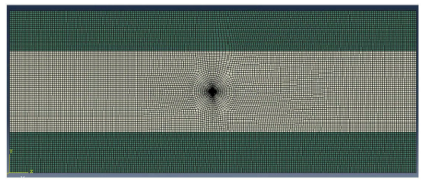Fig.A1   Mesh diagram of the structure without cohesive elements

### 图A2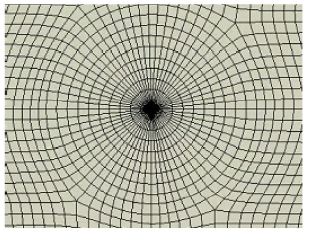Fig.A2   Crack tip grid of the structure without cohesive elements

### 图A3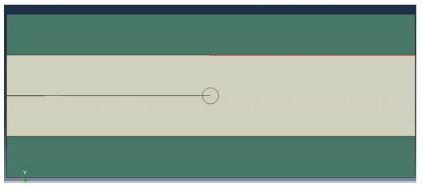Fig.A3   Location of the cohesive elements (orange line)

### 图A4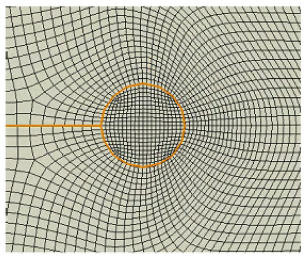Fig.A4   Crack tip grid of the structure with cohesive elements

## 附录 2 MATLAB 求解代码

1. 扫描二维码即可查看具体的 MATLAB 求解代码.2. 或登陆 https://github.com/zff19 查看其中的 MATLAB code 文件即可找到对应 MATLAB 求解代码.

## 参考文献 原文顺序 文献年度倒序 文中引用次数倒序 被引期刊影响因子

Marzi S, Biel A, Stigh U.

On experimental methods to investigate the effect of layer thickness on the fracture behavior of adhesively bonded joints

URL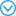A number of different experimental methods are used at two independent laboratories to evaluate the influence of layer thickness on the fracture properties atone batch of a crash resistant epoxy adhesive. Both mode I and II are considered. Novel, as well as state of the art methods are used. In mode I, the Double Cantilever Beam (DCB) and two versions of the Tapered Double Cantilever Beam (TDCB) specimens are utilized; in mode II, two versions of the End Notch Flexure (ENF) and the End-Loaded Shear Joint (ELSJ) specimens are used. Good agreement between the test results is achieved for thin layers in both fracture modes. For thicker layers the variation is larger. (C) 2011 Elsevier Ltd.

Carlberger T, Stigh U.

Influence of layer thickness on cohesive properties of an epoxy-based adhesive—an experimental study

Lee DB, Ikeda T, Miyazaki N, et al.

Effect of bond thickness on the fracture toughness of adhesive joints

Journal of Engineering Materials and Technology, 2004,126(1):14-18

Ranade SR, Guan Y, Ohanehi DC, et al.

A tapered bondline thickness double cantilever beam (DCB) specimen geometry for combinatorial fracture studies of adhesive bonds

URLThe characterization of fracture energy (G(IC)) of an adhesive joint as a function of bondline thickness requires multiple specimens covering a range of bondline thicknesses. In this work, DCB specimens with linearly increasing or decreasing bondline thickness were studied for their feasibility to determine fracture energy as a function of bondline thickness. In a combinatorial characterization sense, this approach explores the possibility to characterize the effect of bondline thickness on fracture energy through fewer tests than those required for a "one at a time" characterization approach, thus offering a significant reduction in characterization times. Fracture energies were characterized under mode I loading conditions using corrected beam theory. The results obtained from linearly increasing or decreasing bondline thickness specimens showed good agreement with those obtained from specimens with a range of constant bondline thicknesses. (C) 2014 Elsevier Ltd.

Cooper V, Ivankovic A, Karac A, et al.

Effects of bond gap thickness on the fracture of nano-toughened epoxy adhesive joints

Polymer, 2012,53(24):5540-5553

URLThe current work is a combined experimental-numerical study of the fracture behaviour of a nano-toughened, structural epoxy adhesive. The mode I fracture toughness of the adhesive is measured using tapered double-cantilever beam (TDCB) tests with various bond gap thicknesses ranging from 0.25 mm to 2.5 mm. Circumferentially deep-notched tensile specimens are independently employed to measure the cohesive strength of the adhesive as a function of constraint. The experimental TDCB test results are predicted numerically for each bond gap thickness using the Finite Volume method and a Dugdale cohesive zone model. A unique relationship between the fracture energy and the constraint level is established. The effect of bond gap thickness on the fracture behaviour of TDCB joints is hence directly attributed to the variation of the intrinsic fracture energy with constraint and not to the variation of the 'far field' plastic zone size with bond gap thickness. Using the well known Rice and Tracey void growth model, a link is established between the voids observed in the fracture process zone, the constraint imposed by the thickness of the adhesive and the resulting fracture energy. (C) 2012 Elsevier Ltd.

Fernandes RL, Freitas ST, Budzik MK, et al.

Engineering Fracture Mechanics, 2019,218:601-607

Akpinar S, Doru MO, Özel A, et al.

The effect of the spew fillet on an adhesively bonded single-lap joint subjected to bending moment

Composites Part B Engineering, 2013,55:55-64

Da Silva LF, Lopes MJC.

Joint strength optimization by the mixed-adhesive technique

URL## Abstract

Cheng J, Taheri F.

A smart single-lap adhesive joint integrated with partially distributed piezoelectric patches

International Journal of Solids and Structures, 2006,43(5):1079-1092

Mao J, Nassar S, Yang X.

An improved model for adhesively bonded DCB joints

Journal of Adhesion Science and Technology, 2014,28(6):613-629

URLAn improved analytical model is proposed for characterizing the fracture behavior of an adhesively bonded double cantilever beam joint under Mode I loading. Novel interfacial normal stress distribution function is used with a key parameter c that is determined using continuum mixture theory. In addition to the mechanical and sectional properties of the adherends, crack length, and overlap area, the model also incorporates the adhesive thickness and material properties as well as the crack tip rotation. Model prediction of the fracture toughness of the joint is entered into finite element analysis to simulate crack propagation under peel loading. The effect of various parameters on the joint fracture properties is discussed. Results show that the proposed model provides better correlation with published experimental data.

Biel A, Stigh U.

Effects of constitutive parameters on the accuracy of measured fracture energy using the DCB-specimen

Engineering Fracture Mechanics, 2008,75(10):2968-2983

URL## Abstract

Several methods exist to estimate the fracture energy for adhesive joints using the double cantilever beam specimen and linear elastic fracture mechanics. Since the mechanical properties of all adhesives are non-linear, errors are generated. By use of an exact solution experiments are simulated. These are evaluated with eight different methods. The influence of the constitutive parameters is systematically studied. This influence is small for most methods. The error due to the choice of evaluation method is considerably larger. One of the commonly used methods gives accurate results; the error is less than 3%. However, most methods yield substantial errors.

Yoshihara H.

Simple estimation of critical stress intensity factors of wood by tests with double cantilever beam and three-point end-notched flexure

Holzforschung, 2007,61(2):182-189

Tamuzs V, Tarasovs S, Vilks U.

Delamination properties of translaminar-reinforced composites

Composites Science and Technology, 2003,63(10):1423-1431

URL## Abstract

In the paper the delamination crack propagation is investigated in 3D woven carbon and glass fiber composites. Composites were produced in 3TEX company and had volume fraction of transverse fibers equal 2–5% of total volume of fibers. The reinforcement acts as crack bridging, greatly improving material resistance to delamination. In this paper investigation of this material has been performed using double cantilever beam technique and models elaborated for large scale bridging in UD composites. The following alterations and novelties are introduced: 1) The appropriate form and shape of specimens with glued additional metallic tabs was elaborated allowing to avoid the premature break of specimen arms; 2) Original loading device was designed to transfer high load directly to crack faces, and additional measurement of crack opening was proposed (as in the case of UD composite) to find the bridging law; 3) The convenient analytical formula for GIc calculation was proposed and used; 4) Instead of traditional R curve which is geometry dependent it was proposed to characterize the delamination resistance as function of crack opening displacement. The quantitative delamination characteristics for materials investigated are presented.

Biel A, Stigh U.

An analysis of the evaluation of the fracture energy using the DCB-specimen

Archives of Mechanics, 2007,59(4-5):311-327

Williams J.

The fracture mechanics of delamination tests

The Journal of Strain Analysis for Engineering Design, 1989,24(4):207-214

De Moura MR, Goncalves J.

Crack equivalent concept applied to the fracture characterization of bonded joints under pure mode I loading

Composites Science and Technology, 2008,68(10-11):2224-2230

Xie W, Sitaraman SK.

Investigation of interfacial delamination of a copper-epoxy interface under monotonic and cyclic loading: Experimental characterization

IEEE Transactions on Advanced Packaging, 2003,26(4):447-452

Ouezdou MB, Chudnovsky A, Moet A.

Kanninen M.

An augmented double cantilever beam model for studying crack propagation and arrest

International Journal of Fracture, 1973,9(1):83-92

A closed form solution for the energy release rate of the double cantilever beam specimen with an adhesive layer

Journal of Composite Materials, 1993,27(4):383-407

Krenk S.

Energy release rate of symmetric adhesive joints

Engineering Fracture Mechanics, 1992,43(4):549-559

Gilchrist M, Smith R.

Fatigue growth of cohesive defects in T-peel joints

Gilchrist M, Smith R.

Static strength and fatigue performance of aluminium-adhesive T-peel joints

Journal of Aerospace Engineering, 1994,208(1):33-40

Palazotto AN, Birman V.

Review of fracture in adhesive joints considering rocket motor application

Journal of Spacecraft and Rockets, 1995,32(3):538-544

Abou-Hamda M, Megahed M, Hammouda M.

Fatigue crack growth in double cantilever beam specimen with an adhesive layer

Engineering Fracture Mechanics, 1998,60(5-6):605-614

Ashcroft I, Hughes D, Shaw S.

Al-Khanbashi A, El-Said EM.

Effect of bond thickness on creep lifetime of adhesive joints under mode I

Journal of Adhesion Science and Technology, 2005,19(7):595-610

Banea MD, Da Silva LFM, Campilho RD.

The effect of adhesive thickness on the mechanical behavior of a structural polyurethane adhesive

Cabello M, Zurbitu J, Renart J, et al.

A general analytical model based on elastic foundation beam theory for adhesively bonded DCB joints either with flexible or rigid adhesives

International Journal of Solids and Structures, 2016,94:21-34

Biel A, Stigh U.

Cohesive zone modelling of nucleation, growth and coalesce of cavities

International Journal of Fracture, 2017,204(2):159-174

/

 〈〉